# Drop specific rows from multiindex Pandas Dataframe

• Difficulty Level : Expert
• Last Updated : 23 May, 2021

In this article, we will learn how to drop specific rows from the multi-index DataFrame.

First, let us create the multi-index DataFrame. The steps are given below:

## Python3

 `import` `numpy as np``import` `pandas as pd`` ` `mldx_arrays ``=` `[np.array([``'lion'``, ``'lion'``, ``'lion'``, ``'bison'``,``                         ``'bison'``, ``'bison'``, ``'hawk'``, ``'hawk'``,``                         ``'hawk'``]),``                ` `               ``np.array([``'height'``, ``'weight'``, ``'speed'``,``                         ``'height'``, ``'weight'``, ``'speed'``,``                         ``'height'``, ``'weight'``, ``'speed'``])]`` ` `# creating a multi-index dataframe``# with random data``multiindex_df ``=` `pd.DataFrame(``  ``np.random.randn(``9``, ``4``), index``=``mldx_arrays,``  ``columns``=``[``'Type A'``, ``'Type B'``, ``'Type C'``, ``'Type D'``])`` ` `multiindex_df.index.names ``=` `[``'level 0'``, ``'level 1'``]``multiindex_df`

Output: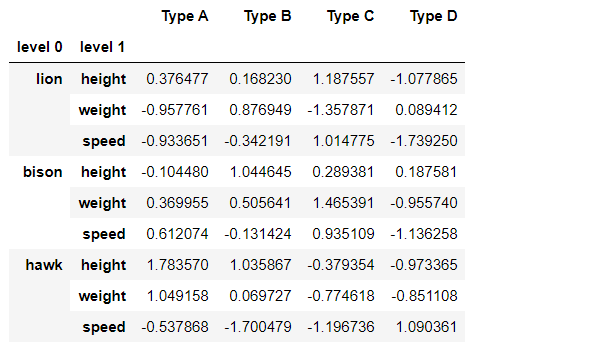Now, we have to drop some rows from the multi-indexed dataframe. So, we are using the drop() method provided by the pandas module. This function drop rows or columns in pandas dataframe.

Syntax: df.drop(‘labels’, level=0, axis=0, inplace=True)

Parameters:

• labels: the parameter mentioned in quotes is the index or column labels to drop
• axis : parameter to drop labels from the rows(when axis=0 or ‘index’) / columns (when axis=1 or ‘columns’).
• level: parameter indicates the level number like 0,1 etc to help identify and manipulate a particular level of data in a multi-index dataframe. For example, there are two levels in the given examples i.e level 1 and level 2.
• inplace: parameter to do operation inplace and return nothing if its value is given True. Here in all the examples, the value of         inplace is given True so that it does the operation and then return nothing.

Example 1: To drop the rows containing ‘lion’ in level 0.

Here ‘lion’ is the label name we want to drop,

• level=0, axis=0 as which depicts rows will be targeted for deletion,
• inplace=True inside the df.drop() function so that it performs the task and returns nothing.

## Python3

 `multiindex_df.drop(``'lion'``, level``=``0``, axis``=``0``, inplace``=``True``)``multiindex_df`

Output: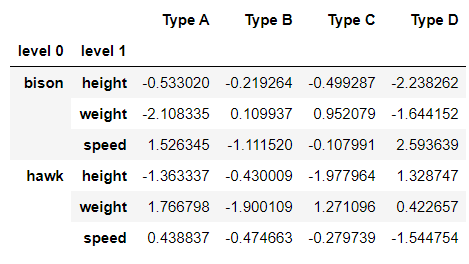Example 2: To drop the rows in level 1 containing ‘weight’.

Here ‘weight’ is the label name we want to drop in level 1 from each of the rows in level 0,

• level=1, axis=0 as which depicts rows will be targeted for deletion,
• inplace=True inside the df.drop() function so that it performs the task and return nothing.

## Python3

 `multiindex_df.drop(``'weight'``, level``=``1``, axis``=``0``, inplace``=``True``)``multiindex_df`

Output: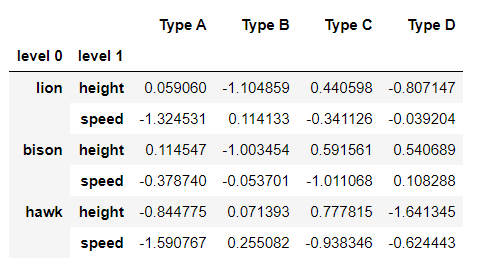Example 3: To drop a single row having a label as ‘weight’ in level 1 inside ‘bison’ in level 0.

Here (‘bison’, ‘weight’) are the label names we want to drop from level 0 and 1 respectively. It simply means that only the row from label ‘bison’ in level 0, the label ‘weight’ from level 1 will be deleted. No need to mention the level as it involves two levels, so only the label names within quotes will work fine,

• axis=0 as which depicts rows will be targeted for deletion
• inplace=True inside the df.drop() function so that it performs the task and return nothing.

## Python3

 `multiindex_df.drop((``'bison'``, ``'weight'``), axis``=``0``, inplace``=``True``)``multiindex_df`

Output: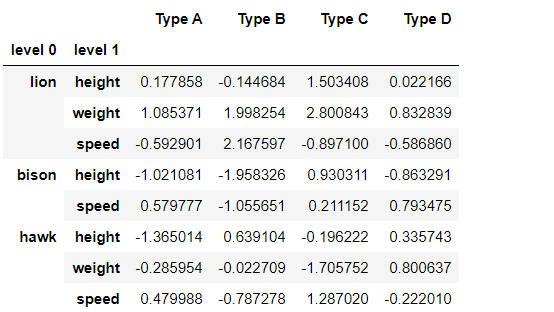Example 4: To drop two rows from level 0.

Here (‘bison’, ‘hawk’) are the label names we want to drop from level 0 which contains multiple rows from level 1. So deletion of the rows from level 0 will result in the dropping of the respective rows from level 1 also.

• axis=0 as which depicts rows will be targeted for deletion,
• inplace=True inside the df.drop() function so that it performs the task and returns nothing.

## Python3

 `multiindex_df.drop([``'bison'``, ``'hawk'``], axis``=``0``, inplace``=``True``)``multiindex_df`

Output 4: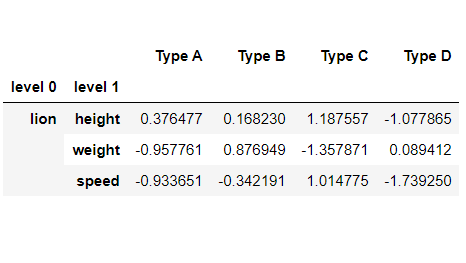My Personal Notes arrow_drop_up